# Exponential mapping

(diff) ← Older revision | Latest revision (diff) | Newer revision → (diff)
A mapping of the tangent space of a manifoldinto. It is defined by a connection given onand is a far-reaching generalization of the ordinary exponential function regarded as a mapping of a straight line into itself.
1) Letbe a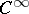-manifold with an affine connection, letbe a point in, let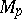be the tangent space toat, letbe a non-zero vector in, and let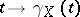be the geodesic passing throughin the direction of. There is an open neighbourhoodof the pointinand an open neighbourhoodofinsuch that the mappingis a diffeomorphism of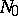onto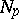. This mapping is called the exponential mapping atand is denoted by. A neighbourhoodis called normal if: 1) the mappingmapsontodiffeomorphically; and 2)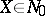and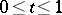imply that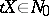. In this caseis said to be a normal neighbourhood of the pointin the manifold. Everyhas a convex normal neighbourhood: Any two points of such a neighbourhood can be joined by exactly one geodesic segment lying in. Ifis a complete Riemannian manifold, thenis a surjective mapping ofonto.
2) Letbe a Lie group with identityand letbe the corresponding Lie algebra consisting of the tangent vectors toat. For every vector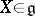there is a unique differentiable homomorphismof the groupintosuch that the tangent vector toatcoincides with. The mapping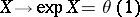is called the exponential mapping of the algebrainto the group. There is an open neighbourhoodof the pointinand an open neighbourhood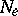ofinsuch that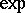is a diffeomorphism ofonto. Letbe some basis for the algebra. The mappingis a coordinate system on; these coordinates are called canonical.
The concept of an exponential mapping of a Lie groupcan also be approached from another point of view. There is a one-to-one correspondence between the set of all affine connections onthat are invariant relative to the group of left translations and the set of bilinear functions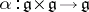. It turns out that the exponential mappingof the algebrainto the groupcoincides with the mappingof the tangent space ofinto the manifoldat the pointin this manifold with respect to the left-invariant affine connection corresponding to any skew-symmetric bilinear function.